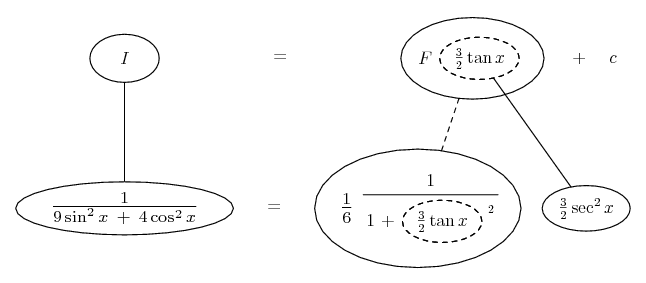# Thread: Solve The Following Trigonometric Integral. (2)

1. ## Solve The Following Trigonometric Integral. (2)

Hello

Solve
$\displaystyle \int \frac{dx}{9sin^2(x)+4cos^2(x)}$

The substitution $\displaystyle u=tan(\frac{x}{2})$ maybe will solve it.
since it is used for the rational trigonometric function, especially which invloves sine and cosine.
But it changes it to a complicated rational function in u.

I believed, there is an easy way !

2. $\displaystyle \frac{1}{9 \sin^2 x + 4 \cos^2 x}$

$\displaystyle =\ \ \frac{1}{4}\ \ \frac{1}{\frac{9}{4} \sin^2 x + \cos^2 x}$

$\displaystyle =\ \ \frac{1}{6}\ \ \frac{\frac{3}{2} \sec^2 x}{\frac{9}{4} \tan^2 x + 1}$

$\displaystyle =\ \ \frac{1}{6}\ \ \frac{1}{(\frac{3}{2} \tan x)^2 + 1}\ \ \frac{3}{2} \sec^2 x$

Edit:

Just in case a picture helps...... where... is the chain rule. Straight continuous lines differentiate downwards (integrate up) with respect to x, and the straight dashed line similarly but with respect to the dashed balloon expression (the inner function of the composite which is subject to the chain rule).

__________________________________________

Don't integrate - balloontegrate!

Balloon Calculus; standard integrals, derivatives and methods

Balloon Calculus Drawing with LaTeX and Asymptote!

#### Search Tags

integral, solve, trigonometric